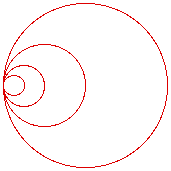# Summing Circles

The largest circle has an area of one. Each following circle has a diameter of 1/2 the next larger circle and shares a common point with all of the remaining circles. If the pattern continues forever and you use infinitesimally small circles, then what is the cumulative area of the all of the circles?

What is the cumulative area of the 1st, 3rd, 5th, etc (odd) circles?

What is the cumulative area of the 2nd, 4th, 6th, etc (even) circles?